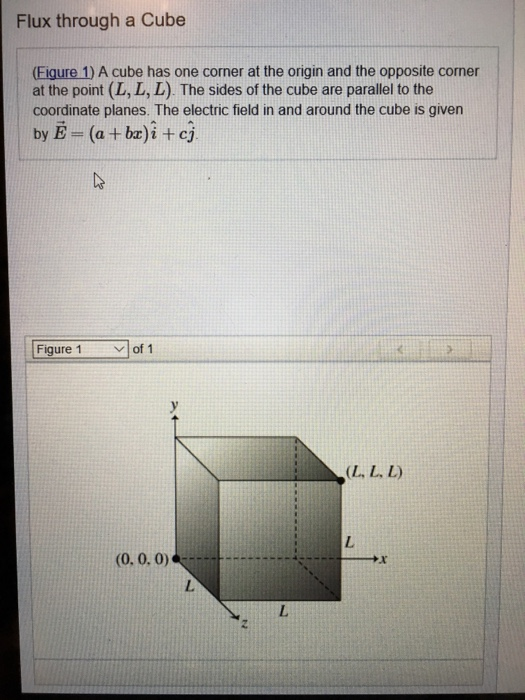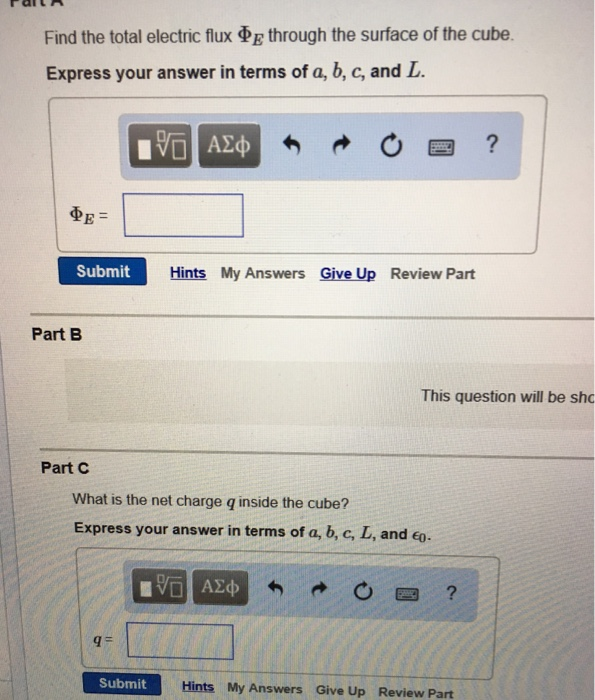Ask question

# Write inequalities to describe the sets The solid cube in the first octant bounded by the coordinate planes and the planes x = 2, y = 2, and z = 2# Write inequalities to describe the sets The solid cube in the first octant bounded by the coordinate planes and the planes x = 2, y = 2, and z = 2

Question
Negative numbers and coordinate planeasked 2021-02-24
Write inequalities to describe the sets The solid cube in the first octant bounded by the coordinate planes and the planes x = 2, y = 2, and z = 2

## Answers (1)2021-02-25
The solid cube (or region) in the first octant bounded by the coordinate plane annd the planes x=a,y=b and z=c is
$$\displaystyle{0}\le{x}\le{a},{0}\le{y}\le{b}{\quad\text{and}\quad}{0}\le{z}\le{c}.$$ The solid cube (or region) in the first octant bounded by the coordinate planes and the planes x=2,y=2 and z=2 is
$$\displaystyle{0}\le{x}\le{2},{0}\le{y}\le{2}{\quad\text{and}\quad}{0}\le{z}\le{2}.$$

### Relevant Questionsasked 2021-02-24
Find the average value of F(x, y, z) over the given region. F(x, y, z) = x2 + 9 over the cube in the first octant bounded by the coordinate planes and the planes x = 2, y = 2, and z =2.asked 2020-11-27
Obtain the volume of the solid which is bounded by a circular paraboloid $$\displaystyle{z}={x}^{{2}}+{y}^{{2}}$$, cylinder $$\displaystyle{x}^{{2}}+{y}^{{2}}={4}$$, and Coordinate plane. And the solid is in the $$\displaystyle{\left({x}\ge{0},{y}\ge{0},{z}\ge{0}\right)}$$.asked 2021-01-13
Average value over a multivariable function using triple integrals. Find the average value of $$\displaystyle{F}{\left({x},{y},{z}\right)}={x}^{{2}}+{y}^{{2}}+{z}^{{2}}$$ over the cube in the first octant bounded bt the coordinate planes and the planes x=5, y=5, and z=5asked 2021-02-25
Determine the volume of the largest box in the first octant with three in the coordinate planes, one vertex at the origin, and its opposite vertex in the plane x+3y+5z=15asked 2021-04-25
Flux through a Cube (Eigure 1) A cube has one corner at the origin and the opposite corner at the point (L, L, L). The sides of the cube are parallel to the coordinate planesasked 2021-01-28
The volume of the largest rectangular box which lies in the first octant with three faces in the coordinate planes and its one of the vertex in the plane $$\displaystyle{x}+{2}{y}+{3}{z}={6}$$ by using Lagrange multipliers.asked 2021-02-27
Solve the equations and inequalities. Write the solution sets to the inequalities in interval notation. $$\displaystyle{\left({x}^{{2}}-{9}\right)}^{{2}}-{2}{\left({x}^{{2}}-{9}\right)}-{35}={0}$$asked 2020-10-27
Find the points of intersection of the line x = 3+2t, y =5+7t, z = -3+t, that is, I(t) = 3+2t, 5+7t, -3+t, with the coordinate planes.asked 2021-03-05
Solve the equations and inequalities. Write the solution sets to the inequalities in interval notation. $$\displaystyle{9}^{{{2}{m}-{3}}}={27}^{{{m}+{1}}}$$asked 2020-12-28
Use the Divergence Theorem to calculate the surface integral F · dS, that is, calculate the flux of F across S.
$$\displaystyle{F}{\left({x},{y},{z}\right)}={3}{x}{y}^{{2}}{i}+{x}{e}^{{z}}{j}+{z}^{{3}}{k}$$,
S is the surface of the solid bounded by the cylinder $$\displaystyle{y}^{{2}}+{z}^{{2}}={9}$$ and the planes x = −3 and x = 1.
...